American Journal of Modern Physics
Volume 4, Issue 3-1, May 2015, Pages: 44-47

Energy Levels Calculations of24Mg and 25Mg Isotopes using OXBASH Code

Saeed Mohammadi, Zohreh Firozi

Department of Physics, Payame Noor University, Tehran, Iran

Email address: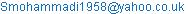(S. Mohammadi)

To cite this article:

Saeed Mohammadi, Zohreh Firozi. Energy Levels Calculations of 24Mg and 25Mg Isotopes using OXBASH Code. American Journal of Modern Physics. Special Issue: Many Particle Simulations. Vol. 4, No. 3-1, 2015, pp. 44-47. doi: 10.11648/j.ajmp.s.2015040301.20

Abstract: In this article by using OXBASH shell model code the energy levels of Magnesium isotopes 24Mg and 25Mg are calculated. This code which is based on one of the most applicable nuclear models, deals with evaluating energy levels. Applying the program for each isotope using the defined codes, introduces several files which each file contains a set of data. Meanwhile, the ground state of excitation energy evaluated by OXBASH code together with energy levels and also probable places for nucleons’ placements in each energy level. Programs will be reliable only when results meet experimental procedures. A compilation of SD-shell energy levels calculated with the USD Hamiltonian and has been published around 1988. A comparison had been made between our results and the available experimental data to test theoretical shell model description of nuclear structure in Magnesium isotopes.  The calculated energy spectrum is in good agreement with the available experimental data.

Keywords: Magnesium Isotopes, OXBASH Code, Shell Model Structure, USD Interaction

Contents

1. Introduction

The nuclear shell model has been very successful in our understanding of nuclear structure: once a suitable effective interaction is found, the shell model can predict various observables accurately and systematically [1-8]. For light nuclei, there are several "standard" effective interactions such as the Cohen-Kurath  and the USD  interactions for the p and SD shells, respectively. Analysis of neutron-rich SD nuclei has been of intense curiosity in recent years as they present new aspects of nuclear structure .Traditional shell-model studies have recently received a renewed interest through large scale shell-model computing in no-core calculations for light nuclei, the 1s0d shell, the 1p0f shell and the 3s2d1g7/2 shell with the inclusion of the 0h11/2 intruder state as well. It is now therefore fully possible to work to large-scale shell-model examinations and study the excitation levels for large systems. In these systems, inter core is assumed and space is determined by considering shell gaps. Figure 1 shows the shell model and some model spaces.

The crucial starting point in all such shell-model calculations is the derivation of an effective interaction, based on a microscopic theory starting from the free nucleon-nucleon (NN) interaction. Although the NN interaction is too short but ﬁnite range, with typical inter particle distances of the order of 1–2 fm, there are indications from both studies of few-body systems and inﬁnite nuclear matter, both real and effective ones, may be of importance. Thus, with many valence nucleons present, such large-scale shell-model calculations may tell us how well an effective interaction which only includes two-body terms reproduces properties such as excitation spectra and binding energies. The problems of deriving such effective operators and interactions are solved in a limited space, the so-called model space, which is a subspace of the full Hilbert space. Several formulations for such expansions of effective operators and interactions exist. For example, for nuclei with 4 < A < 16 p-shell is used of Cohen-Kurath interaction and USD interaction is suitable for 16 < A < 40 SD-shell [9,10].

In order to calculate the nuclear structure properties of both ground and excited states based on the nuclear shell model one needs to have wave functions of those states. These wave functions are obtained by using the shell-model code OXBASH.The code OXBASH for Windows has been used to calculate the nuclear structure for Phosphor nucleus, by employing the SD (independent charges) and SDPN (depending charges) model space with three effective interactions. The first interaction for the lower part of the SD-shell is Chung-Wildenthal particle interaction (CW), secondly, the Universal SD-shell Hamiltonian (USD interaction). In the third interaction the New Universal SD-shell Hamiltonian (USDAPN) is used . Richter et a1 used this shell model successfully in the p-shell, and fp-shell ,  and Wildenthal  and Brown et al in the SD-shell to describe the systematics observed in the spectra and transition strengths. In the present work, we focus our attention on the description of  energy levels of SD shell of Magnesium isotopes 24Mg and 25Mg  which have configurations 0d5/2, 1s1/2and 0d3/2.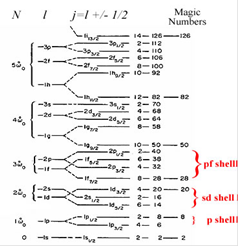Figure 1. p, sd and pf shell model spaces.

2. Theory

One of the approaches to study the structure of a nucleus and NN interactions, named Shell model structure that we deal with all degrees of freedom in this space and consider such all many-body configurations. In this model protons and neutrons move all active single particle orbits with three restrictions, Isospin, Angular momentum and Parity conservation [9, 10]. A J orbit has (2J+1 ) degeneracy for Jz, if we put Nπ protons and Nν neutrons on such orbits, then the numbers of possible configurations are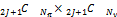. Of course numbers of basis increases in a combinatorial way and the irrelevant numbers must are taken into account.

As is well known, the interaction between two protons, two neutrons or a proton and a neutron is approximately the same,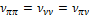, so Isospin (T) was introduced as a new quantum number.

Single-particle wave functions of a neutron and a proton can be expressed with the t = 1/2 spinors .The nuclear states of a nucleus with N neutrons and Z protons (A=N+Z) can be characterized by deﬁnite values of T and MT quantum numbers [1-3]

MT = 1/2(N −Z), 1/2(N −Z) ≤ T ≤ A/2

The configuration for a given nucleus partitioned into core part (Nc, Zc) and an active valance part (N-Nc,Z-Zc). For practical reasons the number of valance nucleons must be small, as the numerical computations increase dramatically in magnitude with this number. Valance nucleons move in a finite number of j-orbits and their Hamiltonian of the valance nucleons is given by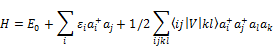Where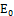is the energy of the inert core,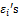are the single particle energies of the valance orbits and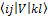are the two-body matrix elements (TBME) of residual interaction amongst the valance particles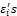effectively take account of interaction between a valance particle and those in the inert core and V is taken from theoretical calculations or phenomenological models. The eigenvectors obtained from H-matrix in turn are used to obtain matrix elements of other physically interesting operators such as electric and magnetic moments, EM transition probabilities, β-decay matrix elements, one- and two-nucleon transfer probabilities, etc.

Finely, the shell-model calculations are confronted with all the available data. A commonly used procedure is to parameterized the effective interactions and even single particle energies of valance orbits and other such operators ( M1, GT, E2 etc.) and then obtain the values of these parameters which give the best numerical fit to the observed set of data points. Computer programs to construct and diagnolize Hamiltonian matrices have been existence for almost 40 years now. An improved modern version ones is OXBASH [11,18] that uses the angular momentum coupled (J) scheme. As the interaction between two valence neutrons, we have to know the set of two-body matrix elements (TBME’s)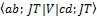with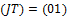OXBASH code only works for Jz=J. By applying J+ operator, it predicts a set of m-scheme vectors that if used for projection will produce a good J-basis. The treatment that follows cannot be generalized for both spin and Isospin to predict exactly a number of m-scheme vectors equal to the good JT-basis dimension. One disadvantage of an m-scheme basis is that it is much larger than the corresponding basis consisting of wave functions coupled to J and T. The n/p formalism enters naturally in the m-scheme formalism, since it only needs to skip those unwanted tz values in each J-orbit in the corresponding SPS_le (Single Particle State _le)

In the second line of approach the two body matrix elements are treated as parameters, and their values are obtained from best fit to experimental data .

Brown and coworkers  have carried extensive studies of energy level and spectroscopic properties of SD-shell nuclei in terms of a unified Hamiltonian applied in full SD-shell model space. The universal Hamiltonian was obtained from a least square fit of 380 energy data with experimental errors of 0.2MeV or less from 66 nuclei. The USD Hamiltonian is defined by 63 SD-shell two body matrix element and their single particle energies. In more recent work Brown and co-workers have modified USD type Hamiltonian to USDA and USDB based on updated set of binding energy and energy levels of O, F, Ne, Na, Mg and P isotopes.

3. OXBASH Code

The calculations have been carried out using the code OXBASH for Windows . The code uses an m-scheme Slater determinant basis and works in the occupation number representation, where the occupancy (vacancy) of a bit in any given position of the computer word symbolizes the presence (absence) of a particle in a specific single particle state (i.e. in a given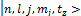state). Using a projection technique, wave functions with good angular momentum J and Isospin T are constructed. The SDPN and SD model spaces consist of (0d5/2, 1s1/2 and 0d3/2) above the Z = 8 and N=8 closed shells for protons and neutrons. CW is an effective interaction that has been used with the SD model space, where the single-particle  energies  are  0.877, -4.15 and -3.28 MeV for subshells 0d3/2, 0d5/2 and 1s1/2, respectively. Also the USD effective interaction has been used with the SD model space, where the single-particle energies are 1.647, -3.948 and -3.164 MeV for subshells 0d3/2, 0d5/2 and 1s1/2, respectively. Meanwhile USDAPN is the effective interaction that has been used, where the single-particle energies for protons and neutrons are 1.980, -3.061 and -3.944 for 0d3/2, 0d5/2 and 1s1/2, respectively .

The OXBASH code uses both m-scheme and jj-coupling. It utilizes a basis of the Slater determinants that are anti-summarized product wave functions. Each of these m-scheme basis states has definite total angular momentum projection quantum number Jz= M and total Isospin projection quantum number Tz. An appropriate expression of the shell-model Hamiltonian is given as the sum of one- and two-body operators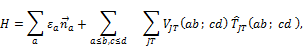Where εa are the single-particle energies,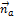is the number operator for the spherical orbit a with quantum number (na ,la ,ja ), VJT(ab cd ) ; is a two-body matrix element, and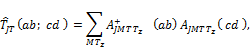is the scalar two-body transition density for nucleon pairs (a, b) and (c, d), each pair coupled to spin quantum numbers JM.

4. Results

The isotopes of Magnesium 24Mg and 25Mg provide a unique laboratory for examining the foundation of SD shell model calculations. The nucleons of the core (16O) are 8 protons and 8 neutrons which are inert in the (1s1/2,0p3/2,0p1/2)J=0,T=0 configuration and the remaining nucleons are distributed over all possible combinations of the 0d5/2,1s1/2 and 0d3/2 orbits according to Pauli Exclusion Principle. The package of program called "SHELL" was used to generate the One Body Density Matrix Element (OBDME), and the package of program called "LPE" is used to calculate the wave functions and energy levels.

We present here some results concerning Ground and excitation energies properties of the Magnesium isotopes for which recent data has been reported in the literature. Table 1 and figure 2 shows data for 24Mg isotope, table 2 and figure 3 shows data for 25Mg isotope. E1 (th) are data calculated by Brown , E3 (th) are data calculated in this work and E2 (exp) are experimental data.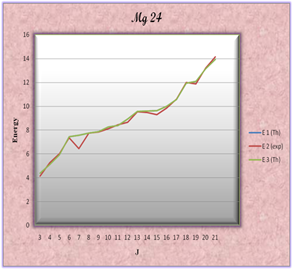Figure 2. Comparison Calculated data with Experimental data for 24Mg.

Table 1. Data for 24Mg. All energies are in MeV.

 J E1(Th) E2(exp) E3(Th) j=2 1 1.509 1.369 1.509 j=2 2 4.122 4.238 4.122 j=4 3 4.378 4.123 4.378 j=3 4 5.097 5.236 5.097 j=4 5 5.934 6.01 5.934 j=2 6 7.442 7.348 7.442 j=0 7 7.561 6.432 7.561 j=1 8 7.764 7.747 7.764 j=5 9 7.883 7.812 7.883 j=6 10 8.263 8.113 8.263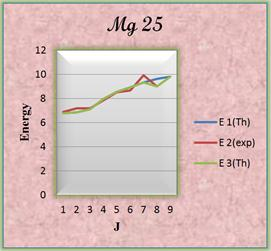Figure 3. Comparison Calculated data with Experimental data for 25Mg.

Table 2. Data for 25Mg. All energies are in MeV.

 J E 1(Th) E 2(exp) E 3(Th) →j=13/21 6.792 6.885 6.796 2→j=7/2 6.875 7.185 6.876 3→j=7/2 7.135 7.181 7.132 4→j=13/2 7.973 7.866 7.973 5→j=15/2 8.574 8.53 8.573 6→j=15/2 8.95 8.656 8.947 7→j=13/2 9.326 9.946 9.334 8→j=13/2 9.634 9.014 9.015 9→j=13/2 9.83 9.836

5. Conclusions

As seen from all figures, very good agreement is obtained for most of energy levels of Magnesium isotopes, and the ordering of levels is correctly reproduced. Regarding to USD data we can judge almost all calculation meet with reasonable success in reproducing the observed level structure.

In general the best and most complete results are found with the largest model space while calculations in an infinite space are not possible and the computation time increases exponentially with model space size so some truncation is required. Also the interaction used must be appropriate for the model space. The empirical interactions are (usually) better determined for smaller model spaces. The model space in OXBASH is defined by the active valance nucleon orbits and our calculated results are reasonably consistent with experimental data, although the structure of odd-even nuclei is much more complicated than their odd-odd neighbours.

References

1. Saeed Mohammadi, Sara Sirjani. Energy Levels Calculations of 29S, 30S and 31S Isotopes. American Journal of Modern Physics. SpecialIssue: Many Particle Simulations. Vol. 4, No. 3-1, 2015, pp. 5-9. doi: 10.11648/j.ajmp.s.2015040301.12
2. S. Mohammadi, S. Arbab, E. Tavakoli. Energy Levels Calculations of 22-23Na and 24-26Mg Isotopes Using Shell Model Code OXBASH. American Journal of Modern Physics. Special Issue: Many Particle Simulations. Vol. 4, No. 3-1, 2015, pp. 27-31. doi: 10.11648/j.ajmp.s.2015040301.16
3. Saeed Mohammadi, Monna Chobbdar. Energy Levels Calculations of 32Cl and 33Cl Isotopes. American Journal of Modern Physics. Special Issue: Many Particle Simulations. Vol. 4, No. 3-1, 2015, pp. 10-14. doi: 10.11648/j.ajmp.s.2015040301.13
4. S. Mohammadi, A. Heydarzade, V. Ragheb. Energy Levels Calculations of 28,30Na and 26,28Al Isotopes Using Shell Model Code OXBASH, American Journal of Modern Physics. Special Issue: Many Particle Simulations. Vol. 4, No. 3-1, 2015, pp. 32-35. doi: 10.11648/j.ajmp.s.2015040301.17
5. S. Mohammadi, F. Bakhshabadi. Calculation of the Energy Levels of Phosphorus Isotopes (A=31 to 35) by Using OXBASH Code. American Journal of Modern Physics. Special Issue: Many Particle Simulations. Vol. 4, No. 3-1, 2015, pp. 15-22. doi: 10.11648/j.ajmp.s.2015040301.14
6. Saeed Mohammadi, Maryam Mounesi. Energy Levels Calculations of 20Ne and 21Ne Isotopes. American Journal of Modern Physics. Special Issue: Many Particle Simulations. Vol. 4, No. 3-1, 2015, pp. 36-39. doi: 10.11648/j.ajmp.s.2015040301.18
7. Saeed Mohammadi, Hassan Rostam Nezhad. Energy Levels Calculations of 26Al and 29Al Isotopes. American Journal of Modern Physics. Special Issue: Many Particle Simulations. Vol. 4, No. 3-1, 2015, pp. 1-4. doi: 10.11648/j.ajmp.s.2015040301.11
8. S. Mohammadi, S. Hesami Rostami, A. Rashed Mohasel and M. Ghamary. Energy Levels Calculations of 36-37-38Ar Isotopes Using Shell Model Code OXBASH. American Journal of Modern Physics. Special Issue: Many Particle Simulations. Vol. 4, No. 3-1, 2015, pp. 23-26.doi: 10.11648/j.ajmp.s.2015040301.15
9. .Nuclear Physics Graduate Course: Nuclear Structure Notes, Oxford, (1980)
10. Effective interactions and the nuclear shell-model, D.J. Dean,T. Engeland M. Hjorth-Jensen, M.P. Kartamyshev, E. Osnes, Progress in Particle and Nuclear Physics 53 419–500(2004)
11. N. A. Smirnova, Shell structure evolution and effective in-medium NN interaction, Chemin du Solarium, BP 120, 33175 Gradignan, France
12. Oxbash for Windows, B. A. Brown, A. Etchegoyen, N. S. Godwin, W. D.M. Rae, W.A. Richter, W.E. Ormand, E.K. Warburton, J.S. Winfield, L. Zhao and C.H. Zimmerman, MSU_NSCL report number 1289.
13. W. A. Richter, M. G. van der Merwe, R. E. Julies, B. A. Brown, Nucl. Phys. A 523,325, (1991)
14. R. E. Julies, PhD. thesis, University of Stelenbosch, (1990).
15. M. G. van der Merwe, PhD. thesis, University of Stelenbosch (1992).
16. B. H. Wildenthal, Prog. Part. Nucl.Phys. 11, 5 (1984).
17. B. A. Brown and B. H. Wildenthal, Ann. Rev. of Nucl. Part. Sci. 38, 29 (1988).
18. http://www.nscl.msu.edu/~brown/
19. B.A. Brown, W.A. Richter, New "USD"Hamiltonians for the sd shell, Physical Review C, 74 (2006)
20. http://www.nscl.msu.edu/~brown/resources/SDE.HTM#a34t2
21. B.H. Wildenthal, Prog. Part. Nucl. Phys. 11, 5 (1984), and references therein
22. M. Homma, B. A. Brown, T. Mizusaki and T. Otsuka, Nucl. Phys.A 704, 134c (2002)
23. B. A. Brown and W. A. Richter, Phys. Rev. C 74, 034315, (2006).

 Contents 1. 2. 3. 4. 5.
Article ToolsAbstractPDF(801K)
Follow on us
PUBLICATION SERVICE
JOIN US
RESOURCES
SPECIAL SERVICES
ADDRESS
Science Publishing Group
548 FASHION AVENUE
NEW YORK, NY 10018
U.S.A.
Tel: (001)347-688-8931